# 4. [Micropython]TPYBoard v202 利用单片机快速实现家庭智能控制平台¶

## 4.4. 0x03 硬件的搭建与连接¶

### 4.4.1. 发光二极管的使用¶### 4.4.2. 直流小电机的使用方法¶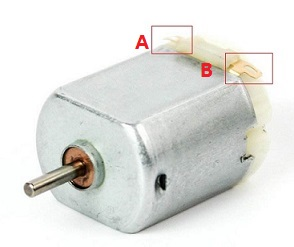### 4.4.3. 三极管S9014（NPN）的使用方法¶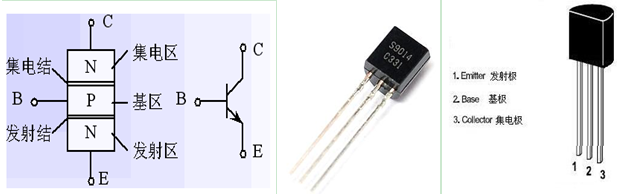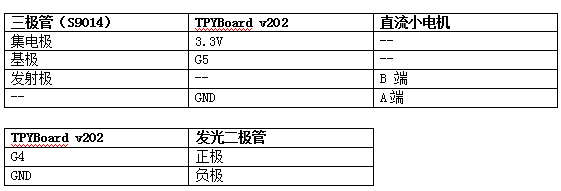## 4.5. 0x04 利用micropython实现web服务器¶

```try:
import usocket as socket
except:
import socket
import network
from machine import UART
from machine import Pin

led_flag=Pin(2, Pin.OUT)#esp8266模块上的小灯 高电平:灭 低电平:亮
led = Pin(4, Pin.OUT)#发光二极管的控制引脚
motor = Pin(5, Pin.OUT)#直流电机的控制引脚
#初始化
led.value(0)
motor.value(0)
led_flag.value(1)
def do_connect(ssid,pwd):
sta_if = network.WLAN(network.STA_IF)#STA 模式
sta_if.active(False)
if not sta_if.isconnected():#判断是否连接
sta_if.active(True)
sta_if.connect(ssid,pwd)#ssid:WIFI名称 pwd:WIFI 密码
while not sta_if.isconnected():
pass
if sta_if.isconnected():
return sta_if.ifconfig()

s = socket.socket()
s.listen(5)
led_flag.value(0)
while True:
res = s.accept()
client_s = res
led_flag.value(1)
while True:
if h == b"" or h == b"\r\n":
break
#print(h)
req+=(h.decode('utf-8'))
print("Request:")
req=req.lower()
req=req.decode('utf-8').split('\r\n')
req_data=req.lstrip().rstrip().replace(' ','')
print(req_data)
if req_data.find('favicon.ico')>-1:
client_s.close()
continue
else:
if len(req_data)<=12:
else:
req_data=req_data.replace('get/?','').replace('http/1.1','')
_name=req_data.find('name')
_pwd=req_data.find('pwd')
if _name>-1 and _pwd>-1:
#判断是否是用户登录
if req_data.find(name)>-1 and req_data.find(pwd)>-1:
s_data=dev_data
else:
f=open('fail.html','r')
f.close()
else:
#判断是否是控制LED
_index=req_data.find('led=')
if _index>-1:
s_data=dev_data
led_val=req_data[_index+4:_index+6].lstrip().rstrip()
print('led:',led_val)
if led_val=='on':
led.value(1)
else:
led.value(0)
print('led:',led.value())
#判断是否是控制电机
_index=req_data.find('motor=')
if _index>-1:
s_data=dev_data
motor_val=req_data[_index+6:_index+8].lstrip().rstrip()
print('motor_val:',motor_val)
if motor_val=='on':
motor.value(1)
else:
motor.value(0)
print('motor:',motor.value())
print('-----------')
client_s.send(s_data)
client_s.close()
led_flag.value(0)

f=open('device.html','r')
f.close()
f.close()
f=open('info.txt','r')
f.close()
name=info.split(',').lstrip().rstrip()
pwd=info.split(',').lstrip().rstrip()
print('name:',name)
print('pwd:',pwd)
print(myip_)
```

info.txt 这里是用文件存放的用户名和密码(英文逗号分隔)，前面是用户名，后面是密码。

## 4.6. 0x05 程序下载测试¶

• 1、使用usb数据线将v202接入到电脑，打开设备管理器，查看加载的端口。我的是COM44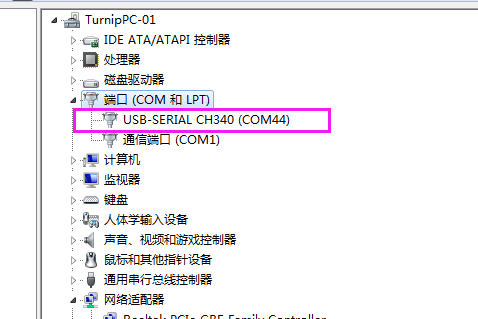※如果驱动安装失败，可以下载CH340的驱动，手动安装。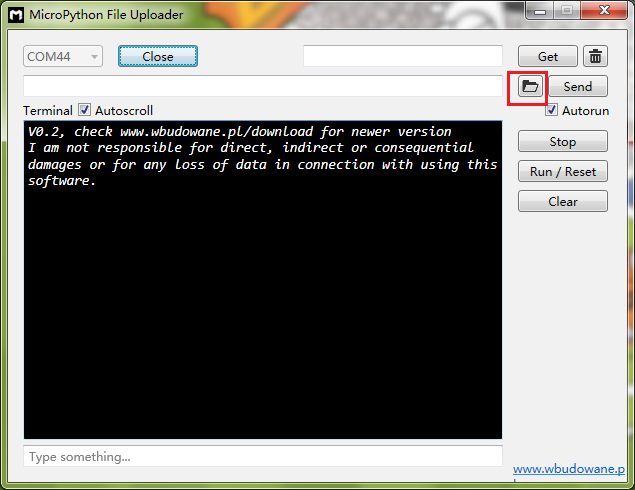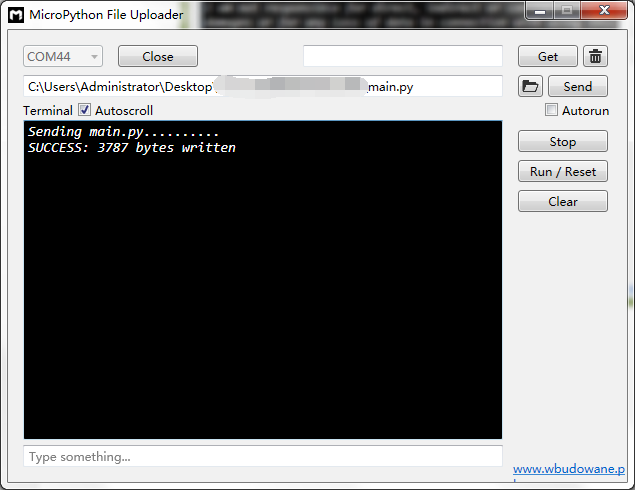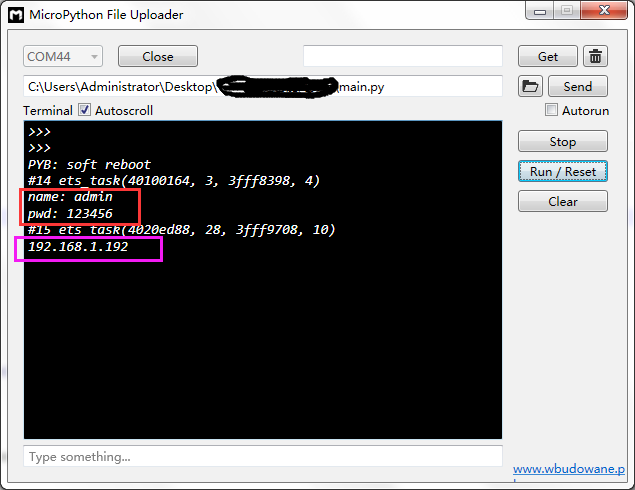## 4.7. 0x06 智能家庭网络平台的使用¶

1、在家庭局域网内，我们可以选用pc或者手机，通过浏览器，打开192.168.1.192 就可以看到登录界面。